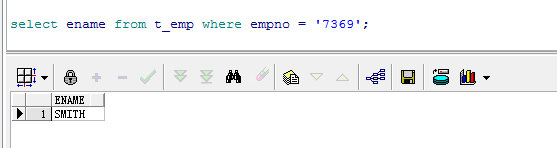Categories

# Oracle SQL tuning of the bind variables usage Introduction

A, SQL execution process Introduction

• 二、绑定变量典型用法

2.1, bind variables in SQL

2.2, using bind variables in PL / SQL

2.3, PL / SQL bind variable quantities

2.4, Java code using bind variables

Recently read “Optimizing Oracle’s SQL-based book,” and made some notes, the author’s personal blog: http: //www.dbsnake.net/

@

A, SQL execution process Introduction

Following Oracle’s cursor on a blog study notes: Oracle Cursor Cursor principle introduction, reintroduction oracle bind variables

Before the introduction of a bind variable, first introduced the concept and execution of SQL hard analysis of:

Sql procedure execution, the text will be sql performs the hash function to obtain a hash value of the object, and then take the hash value Hash Buckets to traverse the cache object handle in the list, find the corresponding cache object handle, then you can get a handle objects in the cache the corresponding sql execution plan, parse trees and other objects, so when the same execution sql second performance will be faster, because there is no analytical obtain the execution plan, parse trees and other objects, if you can not find the library cache object handle, you need to re-analysis, the analytical process too much, easily lead to hard to resolve problems

Hard analysis: is the target in the implementation of Oracle SQL, you can not find in the library cache reuse parse tree and execution plan, and had to start from scratch and build process to resolve the destination SQL respective Parent Cursor and the Child Cursor.
Soft Analysis: Oracle refers to the target in the implementation of SQL, found in the Library Cache in a match of Parent Cursor and Child Cursor, the parse tree and execution plan and stored in the Child Cursor directly take over reuse without parsing from the beginning of the process .

ok, above is a brief introduction to SQL execution process can be seen, if sql execution, execution plan can not be found in the shared pool, parse trees will reproduce parsing sql, execution plan and parse trees, this process is more time-consuming, so try not to find a way to reproduce the parsing sql, need to go directly to the implementation plan has been generated to get a shared pool

For example, select * from sys_user where userid = ‘u10001’; and select * from sys_user where userid = ‘u10002’ ;, these two very similar sql in the implementation process, the resulting execution plan is probably not the same , that is to say after the first sql executed, the second sql continue, if it is found not find the corresponding implementation plan, it will then parse sql, reproduce generate session cursor and a pair of shared cursor (parent cursor and child cursor)

Then, we do not want to re-parsing sql, what method? The method is to use bind variables

Second, the typical use of bind variables

2.1, bind variables in SQL

Typical usage is to use bind variables: variable_name form of, variable_name custom variable name, variabl_name can be a combination of letters, numbers or letters and numbers

OK sql, sql the type of the above, a tape can be used to bind variables represent:

``select * from sys_user where userid = :u;``

So this type of a bunch of sql will only resolve once, not every sql are parsed again, may well increase system capacity

ok, described for example

Preparing the environment:

``````/* 随便建一张表*/
create table t as select * from dba_objects;``````

Note that these scripts can only be executed in PLSQL client sqlplus or command window

``````/* 定义绑定变量vid */
SQL> variable vid number;
/* 给绑定变量赋值为2 */
SQL> exec :vid := 2;``````

PLSQL executed sqlplus client or command window

``````/* 通过绑定变量查询 */
SQL> select * from t where object_id = :vid;``````
`````` /*通过性能视图查询SQL解析情况*/
select a.*, b.name
from v\$sesstat a, v\$statname b
where a.statistic# = b.statistic#
and a.sid = (select distinct sid from v\$mystat)
and b.name like '%parse%';``````
``````/* 去共享池查询一下这种类型的SQL信息*/
select sql_text, parse_calls, executions
from v\$sql
where sql_text like 'select * from t where object_id=%';``````
``````/* 通过共享池查询查询最慢的10条sql*/
SELECT *
FROM (select PARSING_USER_ID,
EXECUTIONS,
SORTS,
COMMAND_TYPE,
sql_text
FROM v\$sqlarea
where ROWNUM < 10;``````

2.2, using bind variables in PL / SQL

``````/* SQL语句使用绑定变量*/
declare
vc_empname varchar2(10);
begin
execute immediate 'select ename from t_emp where empno = :1'
into vc_empname
using 7369;
dbms_output.put_line(vc_empname);
end;
/``````T_emp to write a data table, statistics and whether they were successful, the return value

``````/*DML语句使用绑定变量*/
declare
vc_sql varchar2(2000);
vc_number number;
begin
vc_sql := 'insert into t_emp(empno,ename,job) values(:1,:2,:3)';
execute immediate vc_sql using 7990,'SMITH','HR';
vc_number := sql%rowcount;
dbms_output.put_line(to_char(vc_number));
commit;
end;
/``````

So bind variables core syntax in pl / sql where to:

``  execute immediate [sql语句] using [变量]``

2.3, PL / SQL bind variable quantities

Examples from "SQL Oracle-based optimization," a book, to be achieved is a batch bind variables, fetch key, print out the empno employee information is greater than 7900

``````declare
cur_emp sys_refcursor;
vc_sql varchar2(2000);
type namelist is table of varchar2(10);
enames namelist;
CN_BATCH_SIZE constant pls_integer := 1000;
begin
vc_sql:= 'select ename from t_emp where empno > :1';
open cur_emp for vc_sql using 7900;
loop
fetch cur_emp bulk collect into enames limit CN_BATCH_SIZE;
for i in 1..enames.count loop
dbms_output.put_line(enames(i));
end loop;
exit when enames.count < CN_BATCH_SIZE;
end loop;
close cur_emp;
end;
/
``````

2.4, Java code using bind variables

Do not bind variables wording:

``````String empno = '7369';
String query_sql = 'select ename from t_emp where empno = 7369 ';
stmt = con.prepareStatement( query_sql );
stmt.executeQuery();``````

Use wording bind variables:

``````String empno = 'xxxxx';
String query_sql = 'select ename from t_emp where empno = ? '; //嵌入绑定变量
stmt = con.prepareStatement( query_sql );
stmt.setString(1, empno ); //为绑定变量赋值
stmt.executeQuery();``````

Batch bind variables wording:
This example comes from the "Oracle-based SQL optimization," a book:

``````String vc_sql = 'update t_emp set sal = ? where empno = ?';
pstmt = connection.prepareStatement(dml);
pstmt.clearBatch();
for (int i = 0; i < UPDATE_COUNT; ++ i) {
pstmt.setInt(1, generateEmpno(i));
pstms.setInt(2, generateSal(i));